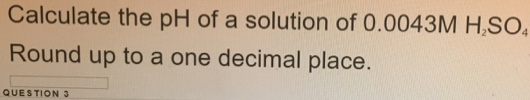# Problem: Calculate the pH of a solution of 0.0043M H2SO4. Round up to a one decimal place.

###### FREE Expert Solution

pH can be computed as the negative logarithm of the H+ molar concentration. In equation, that is:

pH = -log[H+]###### Problem Details

Calculate the pH of a solution of 0.0043M H2SO4. Round up to a one decimal place.